Home MonkeyNotes Printable Notes Digital Library Study Guides Study Smart Parents Tips College Planning Test Prep Fun Zone Help / FAQ How to Cite New Title Request

 Example Use the distance formula to determine if three points A(-5, 3), B(1, -1) and C(4, -3) are collinear. Solution : In D ABC, d (A,C) Ł d (A,B) + d (B, C). . . (by the triangle inequality) and d (A, C) = d (A, B) + d (B, C) if and only if A, B and C are co-linear points. Thus,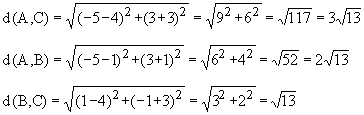Since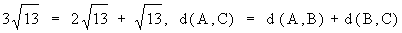\ The three points A, B and C are collinear. Example Graph the equation 2x + y = 7 \ y = -2x + 7 Solution : When x = -1, y = -2 (-1) + 7 i.e. y = 9 When x = 0, y = -2 (0) + 7 i.e. y =7 When x = 1, y = -2 (1) + 7 i.e. y = 5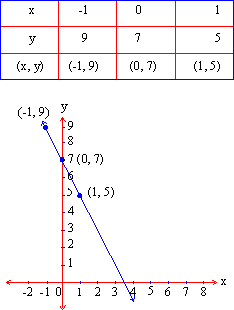Observation : The solution of the equation 2x + y = 7, when plotted, forms a straight line. Conclusion : The equation whose graph is in a straight line is called a linear equation. Thus every first degree equation in x and y of the form ax + by + c = 0 where a, b and c are real numbers, always represents a straight line. There are equations in which a variable is raised to power, shows division by variable, involves variables with square roots, or variables multiplied together. Such equations will not from a straight line. When the solutions are graphed then such equations are called nonlinear equations. Example Graph the equation y = x2 -2 Solution : When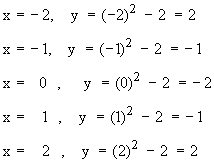i.e.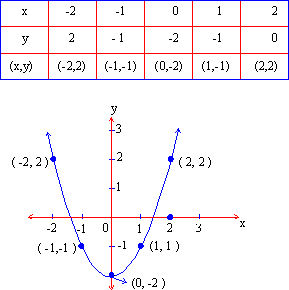From this example, it is clear that solutions of nonlinear equations, when plotted give a curved line. Index 8.1 Definition8.2 Relations, Graphs and Symmetry8.3 Slopes and Intercepts Chapter 9
All Contents Copyright © All rights reserved.
Further Distribution Is Strictly Prohibited.

 Search: All Products Books Popular Music Classical Music Video DVD Toys & Games Electronics Software Tools & Hardware Outdoor Living Kitchen & Housewares Camera & Photo Cell Phones Keywords: